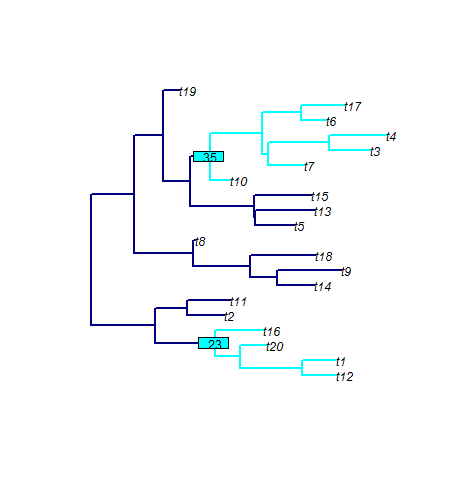# phyloTop

In December 2015 I began maintenance of the package phyloTop for exploring topological properties of trees. It includes functions to calculate tree summary statistics (imbalance, depth, configurations sizes etc.), visualisation tools for highlighting topological properties of a tree in a plot (cherries, subtrees, ladders etc.) and tools for simulating an epidemic and its corresponding transmission genealogy.

It can be found here on CRAN and can be installed and loaded by typing

``````install.packages("phyloTop")

library(phyloTop)
``````

The development version can be found here on GitHub and installed and loaded using

``````devtools::install_github("michellekendall/phyloTop")

library(phyloTop)
``````

Below is an example from the package. The command

``````subtreeShow(rtree(20), nodeList=c(23,35), mainCol="navy",
subtreeCol="cyan", nodeLabelCol="cyan", edge.width=2)
``````

plots a random tree with 20 tips, and highlights in cyan the subclade(s) descending from internal nodes 23 and 35.Updated